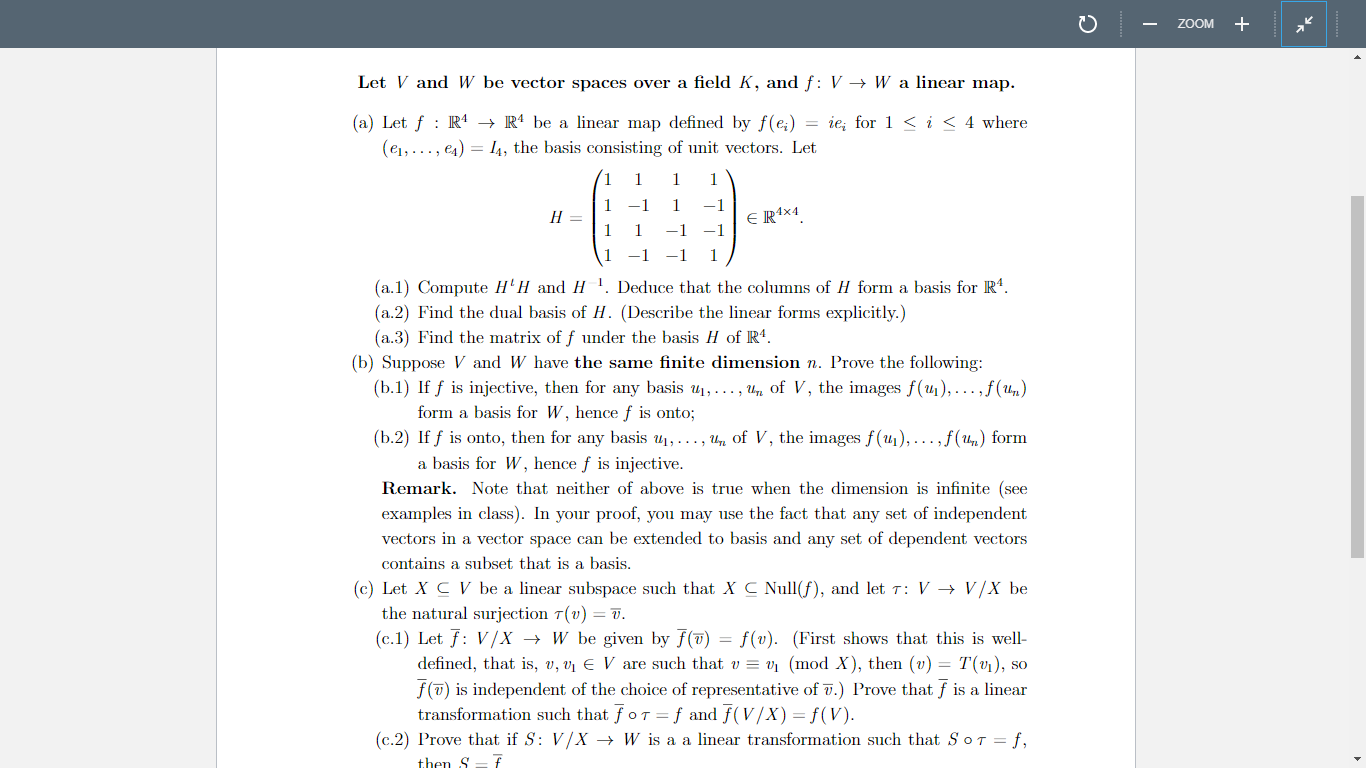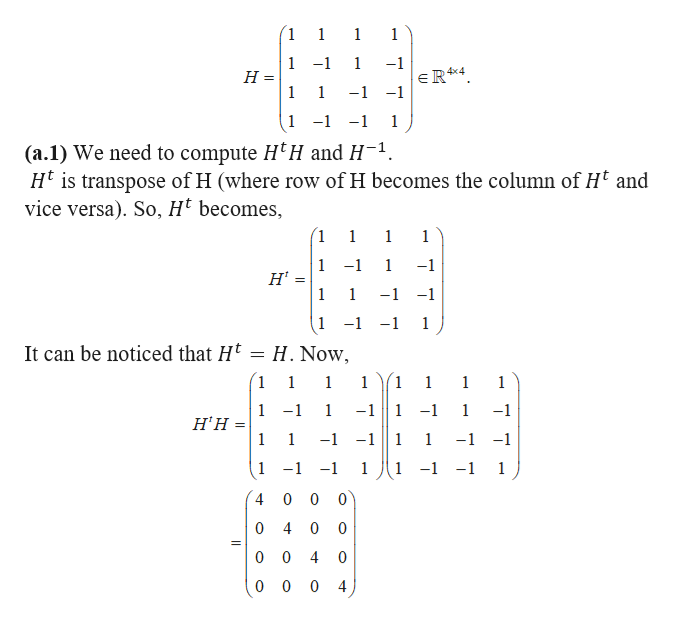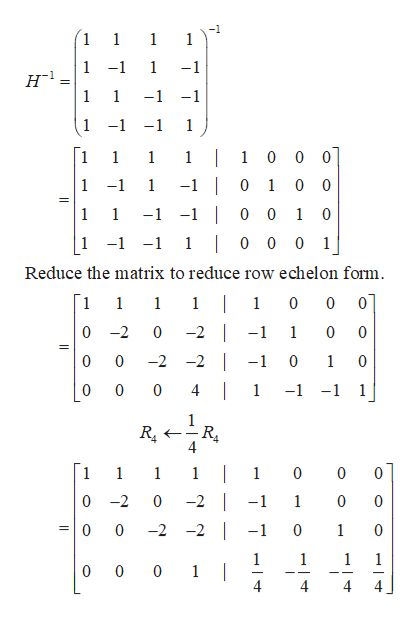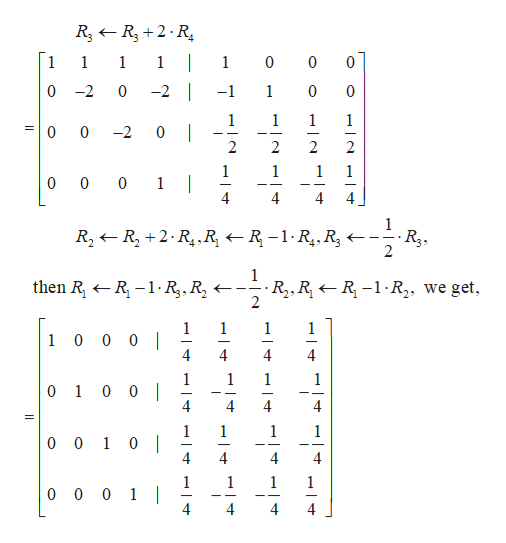ZOOMLet V and W be vector spaces over a field K, and f: V -> W a linear map.(a) Let f R4 -+ R4 be a linear map defined by f(e) = ie; for 1 < i < 4 where(e1,.., e4) I, the basis consisting of unit vectors. Let1111Н —1-11E R4x41-11(a.1) Compute H'H and H. Deduce that the columns of H form a basis for R4(a.2) Find the dual basis of H. (Describe the linear forms explicitly.)(a.3 Find the matrix of f under the basis H of R4(b) Suppose V and W have the same fiite dimension n. Prove the following:(b.1) If f is injective, then for any basis u,..., u of V, the images f(u1),... ,f(1,)form a basis for W, hencef is onto;(b.2) If f is onto, then for any basis u, . .. , u Oof V, the images f(u1), ... ,f(u,) forma basis for W, hence f is injectiveRemark. Note that neither of above is true when the dimension is infinite (seeexamples in class). In your proof, you may use the fact that any set of independentvectors in a vector space can be extended to basis and any set of dependent vectorscontains a subset that is a basis.(c) Let X C V be a linear subspace such that X C Nullf), and let T: V -> V/X bethe natural surjection T(v) = 7.(c.1) Let f V/X > W be given by f(7)defined, that is, v, vi E V are such that u = v, (mod X), then (v) = T(v1), sof(7) is independent of the choice of representative of .) Prove that f is a lineartransformation such that foT f and f(V/X) = f(V)(c.2) Prove that if S: V/X - W is a a linear transformation such that S oT ff(v). (First shows that this is well-then S

Question

Hello, please I need a step by step and a well self-explanatory solution to questions (a.1), (a.2) and (a.3). I don't have a strong background in Algebra. HELP MEhelp_outlineImage TranscriptioncloseZOOM Let V and W be vector spaces over a field K, and f: V -> W a linear map. (a) Let f R4 -+ R4 be a linear map defined by f(e) = ie; for 1 < i < 4 where (e1,.., e4) I, the basis consisting of unit vectors. Let 1 1 1 1 Н — 1 -1 1 E R4x4 1 -1 1 (a.1) Compute H'H and H. Deduce that the columns of H form a basis for R4 (a.2) Find the dual basis of H. (Describe the linear forms explicitly.) (a.3 Find the matrix of f under the basis H of R4 (b) Suppose V and W have the same fiite dimension n. Prove the following: (b.1) If f is injective, then for any basis u,..., u of V, the images f(u1),... ,f(1,) form a basis for W, hencef is onto; (b.2) If f is onto, then for any basis u, . .. , u Oof V, the images f(u1), ... ,f(u,) form a basis for W, hence f is injective Remark. Note that neither of above is true when the dimension is infinite (see examples in class). In your proof, you may use the fact that any set of independent vectors in a vector space can be extended to basis and any set of dependent vectors contains a subset that is a basis. (c) Let X C V be a linear subspace such that X C Nullf), and let T: V -> V/X be the natural surjection T(v) = 7. (c.1) Let f V/X > W be given by f(7) defined, that is, v, vi E V are such that u = v, (mod X), then (v) = T(v1), so f(7) is independent of the choice of representative of .) Prove that f is a linear transformation such that foT f and f(V/X) = f(V) (c.2) Prove that if S: V/X - W is a a linear transformation such that S oT f f(v). (First shows that this is well- then S fullscreen
Step 1

We are given thathelp_outlineImage Transcriptionclose1 1 1 1 1 H = 1 -1 1 -1 4x4 eR4 1 -1 -1 1 -1-1 1 (a.1) We need to compute H'H and H-1 H is transpose of H (where row of H becomes the column of Ht and vice versa). So, H' becomes, 1 111 1 -1 1 -1 H' 1 1 -1 -1 1 -1 -1 1 It can be noticed that H = H. Now 1 1 1 1 1 1 11 -11-1 1 H'H = 1 -1 1 1 -1 -1 -11 1 1 -1 -1 1 -1 1-1-1 1 -1 1 4 0 0 0 4 0 0 0 0 4 10 0 0 0 4 fullscreen
Step 2

Again, we need to find the inverse of H, which is H-1 by Augmented matrix method.help_outlineImage Transcriptionclose-1 1 1 1 1 1 -1 1 -1 н1 1 = 1 -1 -1 1 -1 -1 1 1 0 0 1 0 0 1 1 0 0 1 -1 1 -1 -1 - 0 1 1 0 1 0 -1 1 0 0 0 1 1 -1 Reduce the matrix to reduce row echelon form. 0 | 1 1 1 1 0 0 0 -2 -2 -1 1 10 0 -2 | -2 -1 1 0 | 0 4 1 -1 -1 1 RL 4 | -2 | 1 1 1 1 1 0 0 0 0 -2 0 -1 1 0 0 0 -2 -2 -1 0 1 0 1 0 0 0 1 | 4 1 1 1 4 4 fullscreen
Step 3

Continuing fu...help_outlineImage TranscriptioncloseRsR2 R 1 1 1 1 0 0 1 0 0 -2 0 1 -2 -1 0 1 1 1 1 0 0 -2 0 2 2 2 2 1 0 0 0 1 | 4 1 1 1 4 1 R2R,2.R1 R R-1-R.,, R 1 - R -1R R then R R2,RR-1 R2, we get, 2 1 1 0 0 0 | 1 1 1 4 1 1 0 1 0 0 | 4 4 4 4 = 1 0 0 1 0 || 4 1 1 1 1 0 0 0 1 | 4 4 4 fullscreen

Want to see the full answer?

See Solution

Want to see this answer and more?

Our solutions are written by experts, many with advanced degrees, and available 24/7

See Solution
Tagged in

Math